# PSAT Math : Graphing Quadratics & Polynomials

## Example Questions

2 Next →

### Example Question #11 : Graphing Quadratics & Polynomials

Complete the square to calculate the maximum or minimum point of the given function.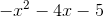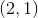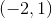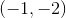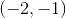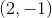Explanation:

Completing the square method uses the concept of perfect squares. Recall that a perfect square is in the form,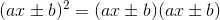where when multiplied out,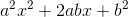the middle term coefficient, when divided by two and squared, results in the coefficient of the last term.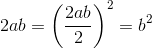Complete the square for this particular function is as follows.First factor out a negative one.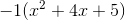Now identify the middle term coefficient.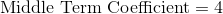Now divide the middle term coefficient by two.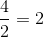From here write the function with the perfect square.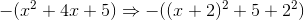When simplified the new function is,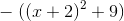Since theterm is negative, the parabola will be opening down. This means that the function has a maximum value at the vertex. To find thevalue of the vertex set the inside portion of the binomial equal to zero and solve.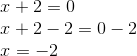From here, substitute the thevalue into the original function.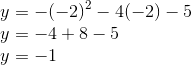Therefore the maximum value occurs at the point.

### Example Question #11 : Calculate Maximum Or Minimum Of Quadratic By Completing The Square: Ccss.Math.Content.Hsa Sse.B.3b

Complete the square to calculate the maximum or minimum point of the given function.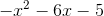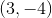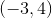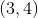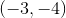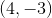Explanation:

Completing the square method uses the concept of perfect squares. Recall that a perfect square is in the form,where when multiplied out,the middle term coefficient, when divided by two and squared, results in the coefficient of the last term.Complete the square for this particular function is as follows.First factor out a negative one.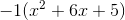Now identify the middle term coefficient.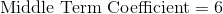Now divide the middle term coefficient by two.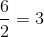From here write the function with the perfect square.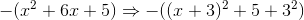When simplified the new function is,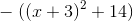Since theterm is negative, the parabola will be opening down. This means that the function has a maximum value at the vertex. To find thevalue of the vertex set the inside portion of the binomial equal to zero and solve.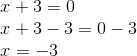From here, substitute the thevalue into the original function.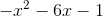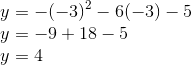Therefore the maximum value occurs at the point.

2 Next →

### All PSAT Math Resources